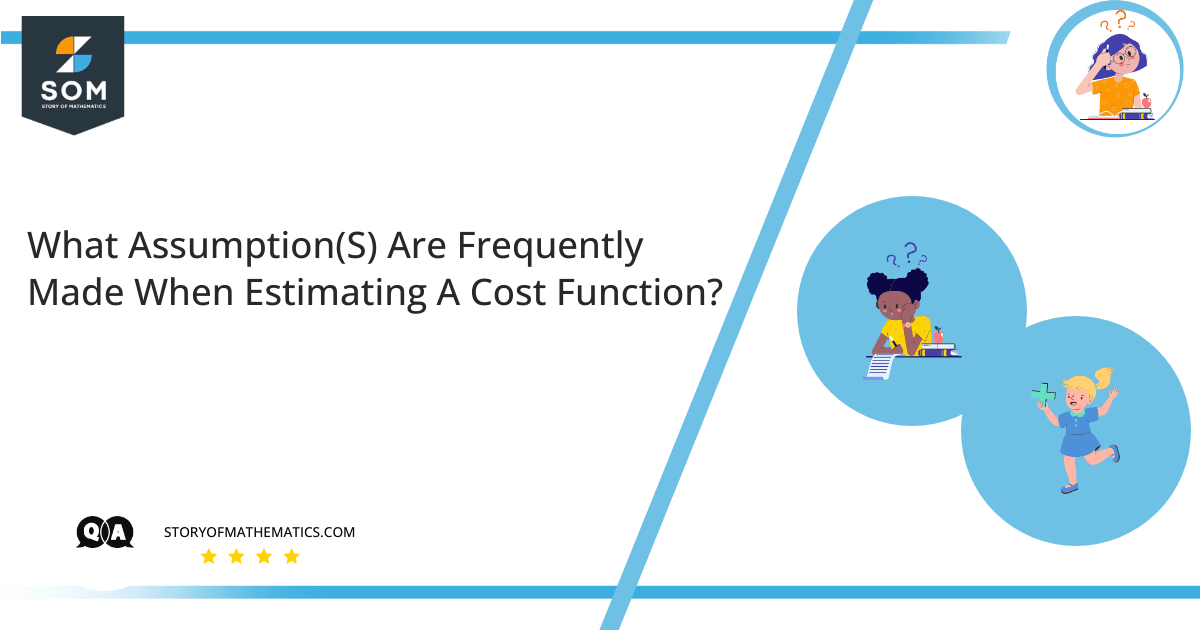# What assumption(s) are frequently made when estimating a cost function?The aim of the question is to find the two assumptions which are considered while estimating the cost function.

The basic concept behind estimating cost function is cost behavior analysis. Cost behavior analysis identifies the change in the total cost of the product at the end due to the change in its activity level. For this purpose mathematical cost functions are used.

There are two types of costs:

1. Variable cost
2. Fixed cost

Here, Fixed cost remains fixed throughout until the end, whereas the variable cost, as the name suggests, varies with change in the activity level.

Cost functions can be represented in the form of an equation or graphical representation for a better understanding. Its representation in the form of an equation includes the variables on which cost function depends, a slight change in these variables thus has a significant effect on the total cost.

We know that the equation for cost function is:

$cost model = fixed cost + variable cost \times x$

It can also be represented as:

$y = ax + h$

where,

$y=$ value at $y-axis$

$a=$ numerical value with axis $x$

$x =$  value at $x-axis$

$h=$ numerical value

To represent a cost function following two points are kept in mind:

1. Changes in the cost function variables depict the change in the final cost.
2. The cost function is considered a linear function.

There are different methods for Cost Analysis, which include,

-Quantitative Analysis

-High-Low Cost Analysis

-Regression Cost Analysis

In quantitative analysis, the easiest method is the high low-cost method. In the high low-cost method, we consider the highest and the lowest costs in the data set to determine the total cost function.

In the Regression Analysis, we not only consider the highest and the lowest values but the rest of the variables as well, and with the help of software such as Microsoft excel we get the required cost function. This method is more accurate and the probability of error is also less.

## Numerical Results

To represent a cost function following two basic points are considered:

1. Changes in the cost function variables depict the change in the final cost.
2. The cost function is considered a linear function.

## Example

A Petroleum industry manager wants to find out the cost analysis equation for the given data set below by applying high-low cost behavior. Find out the full cost function.

Days                  Total overhead cost                 Labor hours

Monday                           $10,000 100 Tuesday$5,000                                  50

Wednesday                        $1500 18 Thursday$8000                                   75

Friday                               $11,000 135 Saturday$2000                                   24

Sunday                              $9000 98 So we gather information for highest and lowest observation: Highest value =$11,000

Highest unit = 135

Lowest value = $1500 Lowest unit = 18 Difference value =$9500

Difference unit = 117

$Slope = \frac {9500}{117} =81.20$

Variable cost per unit = $81.20 fixed cost =$38.46

Equation for cost function is:

$cost\ model = fixed\ cost + variable\ cost\times x$

$cost\ model = 38.46 + 81.20 \times x$